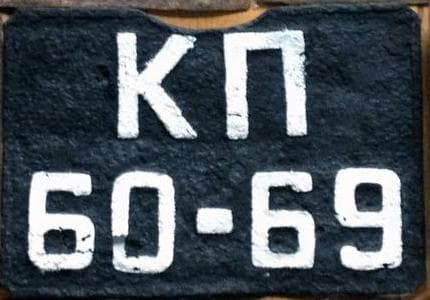johndcook.com Go back Open original

# The Soviet license plate game and Kolmogorov complexity

By JohnPhysicist Lev Landau used to play a mental game with Soviet license plates . The plates had the form of two digits, a dash, two more digits, and some letters.

## Rules of the game

His game was to apply high school math operators to the numbers on both side of the dash so that the dash could be replaced by an equal sign. For example, given the license plate 44-74, one solution would be

4! + 4 = 7*4.

Note that we can insert operators, such as !, +, and *, but not more digits.

Is there a solution for every possible license plate? That depends on what class of operators you allow.

You could trivialize the game by applying the fractional part operation {x} to both sides since the fractional part of an integer is zero. You could disallow the fractional part operator on the grounds that it’s not clearly a high school math operation, or just disallow it because it makes the game uninteresting.

## Universal solution

It turns out there’s a universal solution, starting with the observation that

√(n + 1) = sec arctan √n.

If one side is greater than the other by one, the formula above gives an immediate solution. For example, a solution for the license plate 89-88 would be

√89 = sec arctan √88.

If the difference is greater, the formula can be applied repeatedly. For example, we could apply the formula twice to obtain

√(n + 2) = sec arctan √(n + 1) = sec arctan sec arctan √n

and so a possible solution for 35-37 is

sec arctan sec arctan √35 = √37.

## Kolmogorov complexity

Given that a solution is always possible, we can make the game more interesting by looking for the simplest solution. We have some intuitive idea what this means. With our example of 44-74, the first solution

4! + 4 = 7*4

is simpler than the universal solution

√74 = sec arctan sec arctan … √44.

which would require applying secant and arctangent each 30 times.

The Kolmogorov complexity of an object is the length of the shortest computer program to produce the object. We could compute the Kolmogorov complexity of the functions applied to the digits on each side in order to measure how complex a solution is.

To make this precise, we need to specify what our programming language is, and that’s not as easy as it sounds. If we think of mathematics notation as a programming language, do we want to count ! as one character and arctan as 6 characters? That doesn’t seem right. If we wrote “arctan” as “atn” we would use fewer characters without producing a different solution.

## Complexity of Python code

To make things more objective, we could look at the length of actual computer programs rather than imagining math notation to be a programming language. Say we choose Python. Then here are a couple functions that compute our two solutions for license plate 44-74.

``` from math import sqrt, cos, atan def f(): sec = lambda x: 1/cos(x) y = sqrt(44) for _ in range(30): y = sec(atan(y)) return y def g(): return sqrt(77)
```

We could measure the complexity of our functions `f` and `g` by counting the number of characters in each one. But there still are difficulties.

What about the import statement? It should count toward the length of `f` because it uses everything imported but `g` could have used a shorter statement that only imported `sqrt`. More fundamentally, are we cheating by even importing a library?

Furthermore, the two functions above don’t produce exactly the same output due to limited precision. We could imagine that our imported functions are infinitely precise, but then we’re not really using Python but rather an idealized version of Python.

And what about the loop? That introduced new digits, 3 and 0, and so violates the rules of Landau’s game. So should we unroll the loop before calculating complexity?

## Thought experiment

Kolmogorov complexity is a very useful concept, but it’s more of a thought experiment than something you can practically compute. We can imagine the shortest program to compute something, but we can seldom know that we’ve actually found such a program. All we can know in practice is upper bounds.

In theory you can enumerate all Turing machines of a given length, or all Python programs of a given length, and find the shortest one that does a given task, but the list grows exponentially with length.

However, it is possible to compute the length of particular programs if we deal with some of the complications mentioned above. We could make Landau’s game a two-player game by seeing who could come up with the simpler solution in a fixed amount of time.

## Back to Landau

If we allow sine and degree in our set of operators, there’s a universal solution due to B. S. Gorobets. For n ≥ 6, n! is a multiple of 360, and so

sin (n!)° = 0.

And if n is less than 6, it’s two-digit representation begins with zero, so we can multiply the digits to get zero.

If we disallow transcendental functions, we block Gorobets’ trick and we have functions whose length we can objectively measure in a programming language.

## Related posts

 Harun Šiljak. Soviet Street Mathematics: Landau’s License Plate Game. Mathematical Intelligencer, https://doi.org/10.1007/s00283-017-9743-9

Photo credit CC BY-SA 3.0 via Wikimedia Commons
Русский: Задний автомобильный номер СССР образца 1936 года